DFT’s Part 2: It’s a little complex…

Whole Numbers and Integers

Once upon a time you learned how to count. You were probably taught to count your fingers… 1, 2, 3, 4 and so on. Although no one told you so at the time, you were being taught a set of numbers called whole numbers.

Sometime after that, you were probably taught that there’s one number that gets tacked on before the ones you already knew – the number 0.

A little later, sometime after you learned about money and the fact that we don’t have enough, you were taught negative numbers… -1, -2, -3 and so on. These are the numbers that are less than 0.

That collection of numbers is called integers – all “countable” numbers that are negative, zero and positive. So the collection is typically written

… -5, -4, -3, -2, -1, 0, 1, 2, 3, 4, 5 …

Rational Numbers

Eventually, after you learned about counting and numbers, you were taught how to divide (the mathematical word for “sharing equally”). When someone said “20 divided by 5 equals 4” then they meant “if you have 20 sticks, then you could put those sticks in 4 piles with 5 sticks in each pile.” Eventually, you learned that the division of one number by another can be written as a fraction like 3/1 or 20/5 or 5/4 or 1/3.

If you do that division the old-fashioned way, you get numbers like this:

3 1 = 3.000000000 etc…

20 5 = 4.00000000 etc…

5 4 = 1.200000000 etc…

1 3 = 0.333333333 etc…

The thing that I’m trying to point out here is that eventually, these numbers start repeating sometime after the decimal point. These numbers are called rational numbers.

Irrational Numbers

What happens if you have a number that doesn’t start repeating, no matter how many numbers you have? Take a number like the square root of 2 for example. This is a number that, when you multiply it by itself, results in the number 2. This number is approximately 1.4142. But, if we multiply 1.4142 by 1.4142, we get 1.99996164 – so 1.4142 isn’t exactly the square root of 2. In fact, if we started calculating the exact square root of 2, we’d result in a number that keeps going forever after the decimal place and never repeats. Numbers like this (π is another one…) that never repeat after the decimal are called irrational numbers

Real Numbers

All of these number types – rational numbers (which includes integers) and irrational numbers fall under the general heading of real numbers. The fact that these are called “real” implies immediately that there is a classification of numbers that are “unreal” – but we’ll get to that later…

Imaginary Numbers

Let’s think about the idea of a square root. The square root of a number is another number which, when multiplied by itself is the first number. For example, 3 is the square root of 9 because 3*3 = 9. Let’s consider this a little further: a positive number muliplied by itself is a positive number (for example, 4*4 = 16… 4 is positive and 16 is also positive). A negative number multiplied by itself is also positive (i.e. 4*-4 = 16).

Now, in the first case, the square root of 16 is 4 because 4*4 = 16. (Some people would be really picky and they’ll tell you that 16 has two roots: 4 and -4. Those people are slightly geeky, but technically correct.) There’s just one small snag – what if you were asked for the square root of a negative number? There is no such thing as a number which, when multiplied by itself results in a negative number. So asking for the square root of -16 doesn’t make sense. In fact, if you try to do this on your calculator, it’ll probably tell you that it gets an error instead of producing an answer.

For a long time, mathematicians just called the square root of a negative number “imaginary” since it didn’t exist – like an imaginary friend that you had when you were 2… However, mathematicians as a general rule don’t like loose ends – they aren’t the type of people who leave things lying around… and having something as simple as the square root of a negative number lying around unanswered got on their nerves.

Then, in 1797, a Norwegian surveyor named Casper Wessel presented a paper to the Royal Academy of Denmark that described a new idea of his. He started by taking a number line that contains all the real numbers like this: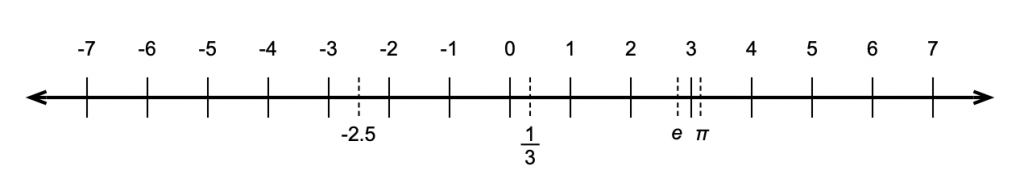Figure 1: The number line containing all real numbers.

He then pointed out that multiplying a number by -1 was the same as rotating by an angle of 180º, like this: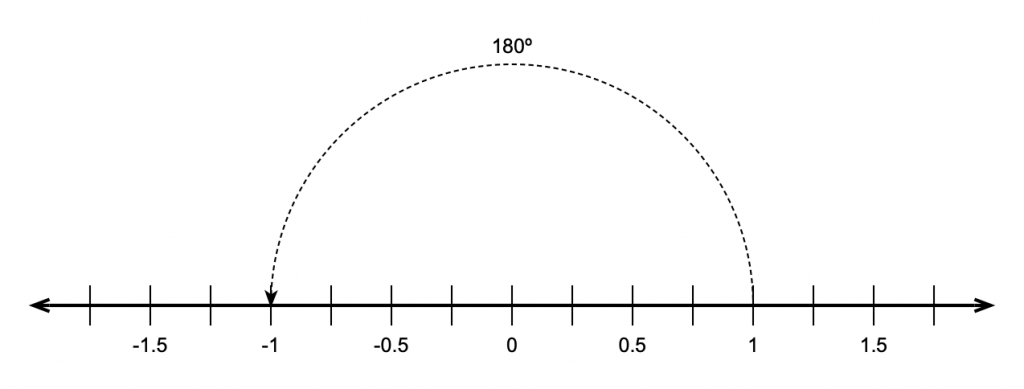Figure 2: Multiplying a number by -1 is the same as rotating by 180º

He then reasoned that, if this were true, then the square root of -1 must be the same as rotating by 90º.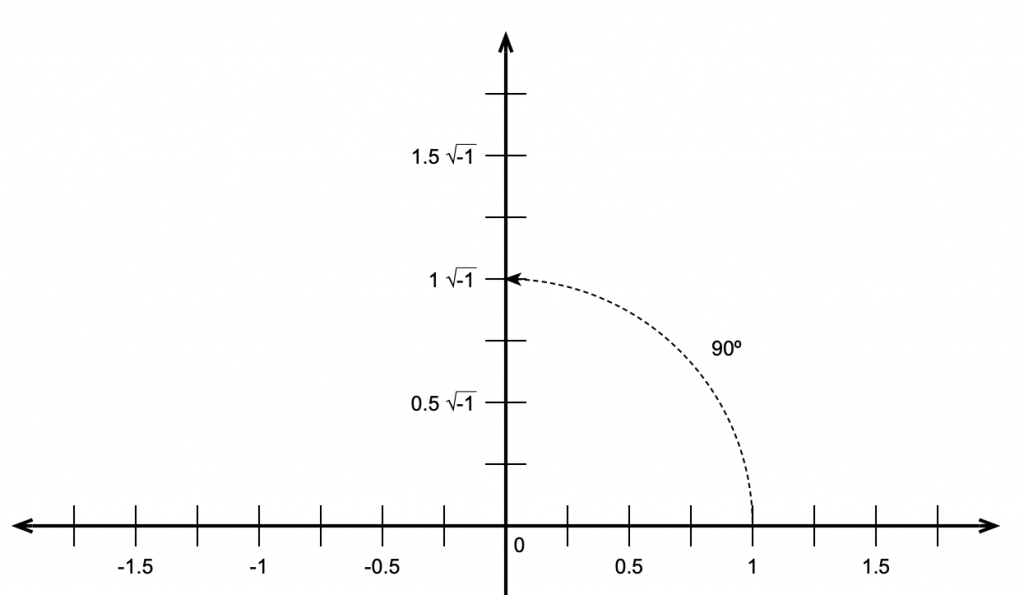Figure 3: This means that the square root of -1 must be the same a rotating by 90º.

This meant that the number line we started with containing the real numbers is the X-axis on a 2-dimensional plane where the Y-axis contains the imaginary numbers. That plane is called the Z plane, where any point (which we’ll call ‘Z’) is the combination of a real number (X) and an imaginary number (Y).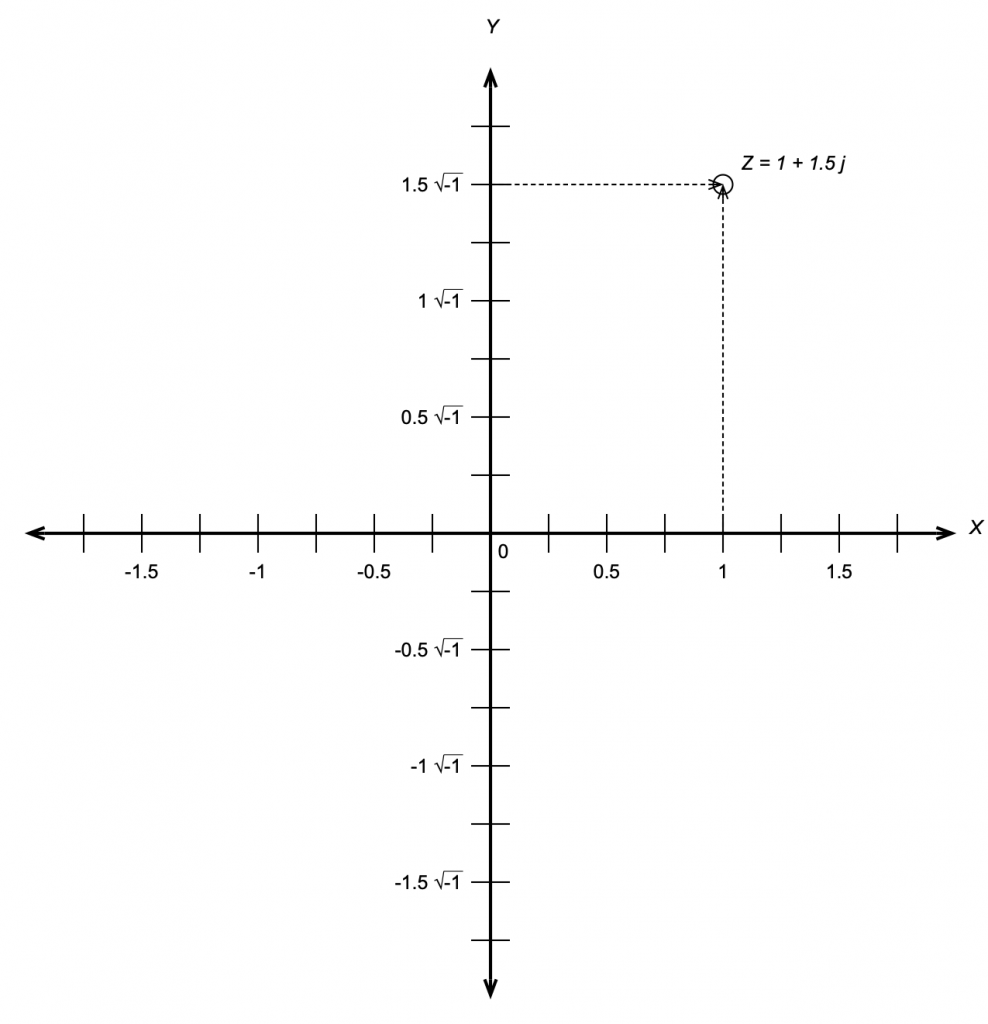Figure 4: The Z-plane, where X-values are real and the Y-values are imaginary.

If you look carefully at Figure 4, you’ll see that I used a “j” to indicate the imaginary portion of the number. Generally speaking, mathematicians use and physicists and engineers use so we’ll stick with j. (The reason physics and engineering people use j is that they use i to mean “electrical current”.)

“What is j?” I hear you cry. Well, is just the square root of -1. Of course, there is no number that is the square root of -1

and therefore

Now, remember that = -1. This is useful for any square root of any negative number, you just calculate the square root of the number pretending that it was positive, and then stick a after it. So, since the square root of 16, abbreviated sqrt(16) = 4 and sqrt(-1) = j, then sqrt(-16) = 4j.

Complex numbers

Now that we have real and imaginary numbers, we can combine them to create a complex number. Remember that you can’t just mix real numbers with imaginary ones – you keep them separate most of the time, so you see numbers like

3+2j

This is an example of a complex number that contains a real component (the 3) and an imaginary component (the 2j). In some cases, these numbers are further abbreviated with a single Greek character, like α or β, so you’ll see things like

α = 3+2j

In other cases, you’ll see a bold letter like the following:

Z = 3+2j

A lot of people do this because they like reserving Greek letters like α and ϕ for variables associated with angles.

Personally, I like seeing the whole thing – the real and the imaginary components – no reducing them to single Greek letters (they’re for angles!) or bold letters.

Absolute Value (aka the Modulus)

The absolute value of a complex number is a little weirder than what we usually think of as an absolute value. In order to understand this, we have to look at complex numbers a little differently:

Remember that j*j = -1.

Also, remember that, if we have a cosine wave and we delay it by 90º and then delay it by another 90º, it’s the same as inverting the polarity of the cosine, in other words, multiplying the cosine by -1. So, we can think of the imaginary component of a complex number as being a real number that’s been rotated by 90º, we can picture it as is shown in the figure below.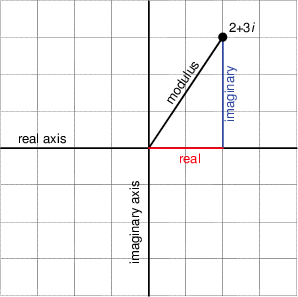Figure 5. The relationship bewteen the real and imaginary components for the number (2 + 3 j). Notice that the X and Y axes have been labeled the “real” and “imaginary” axes.

Notice that Figure 5 actually winds up showing three things. It shows the real component along the x-axis, the imaginary component along the y-axis, and the absolute value or modulus of the complex number as the hypotenuse of the triangle. This is shown in mathematical notation in exactly the same way as in normal math – with vertical lines. For example, the modulus of 2+3j is written |2+3j|

This should make the calculation for determining the modulus of the complex number almost obvious. Since it’s the length of the hypotenuse of the right triangle formed by the real and imaginary components, and since we already know the Pythagorean theorem then the modulus of the complex number (a + b j) is

Given the values of the real and imaginary components, we can also calculate the angle of the hypotenuse from horizontal using the equation

This will come in handy later.

Complex notation or… Who cares?

This is probably the most important question for us. Imaginary numbers are great for mathematicians who like wrapping up loose ends that are incurred when a student asks “what’s the square root of -1?” but what use are complex numbers for people in audio? Well, it turns out that they’re used all the time, by the people doing analog electronics as well as the people working on digital signal processing. We’ll get into how they apply to each specific field in a little more detail once we know what we’re talking about, but let’s do a little right now to get a taste.

In the previous posting, that introduces the trigonometric functions sine and cosine, we looked at how both functions are just one-dimensional representations of a two-dimensional rotation of a wheel. Essentially, the cosine is the horizontal displacement of a point on the wheel as it rotates. The sine is the vertical displacement of the same point at the same time. Also, if we know either one of these two components, we know:

1. the diameter of the wheel and
2. how fast it’s rotating

but we need to know both components to know the direction of rotation.

At any given moment in time, if we froze the wheel, we’d have some contribution of these two components – a cosine component and a sine component for a given angle of rotation. Since these two components are effectively identical functions that are 90º apart (for example, a cossine wave is the same as a sine that’s been delayed by 90º) and since we’re thinking of the real and imaginary components in a complex number as being 90º apart, then we can use complex math to describe the contributions of the sine and cosine components to a signal.

Huh?

Let’s look at an example. If the signal we wanted to look at a signal that consisted only of a cosine wave, then we’d know that the signal had 100cosine and 0sine. So, if we express the cosine component as the real component and the sine as the imaginary, then what we have is:

1 + 0 j

If the signal were an upside-down cosine, then the complex notation for it would be (1 + 0 jbecause it would essentially be a cosine * -1 and no sine component. Similarly, if the signal was a sine wave, it would be notated as (0 1 j).

This last statement should raise at least one eyebrow… Why is the complex notation for a positive sine wave (0 1 j)? In other words, why is there a negative sign there to represent a positive sine component? (Hint – we want the wheel to turn clockwise… and clocks turn clockwise to maintain backwards compatibility with an earlier technology – the sundial. So, we use a negative number because of the direction of rotation of the earth…)

This is fine, but what if the signal looks like a sinusoidal wave that’s been delayed a little? As we saw in the previous posting, we can create a sinusoid of any delay by adding the cosine and sine components with appropriate gains applied to each.

So, is we made a signal that were 70.7sine and 70.7cosine. (If you don’t know how I arrived that those numbers, check out the previous posting.) How would you express this using complex notation? Well, you just look at the relative contributions of the two components as before:

0.707 – 0.707 j

It’s interesting to notice that, although this is actually a combination of a cosine and a sine with a specific ratio of amplitudes (in this case, both at 0.707 of “normal”), the result will look like a sine wave that’s been shifted in phase by -45º (or a cosine that’s been phase-shifted by 45º). In fact, this is the case – any phase-shifted sine wave can be expressed as the combination of its sine and cosine components with a specific amplitude relationship.

Therefore (again), any sinusoidal waveform with any phase can be simplified and expressed as its two elemental components, the gains applied to the cosine (or real) and the sine (or imaginary). Once the signal is broken into these two constituent components, it cannot be further simplified.

Link to DFT’s Part 3: The Math